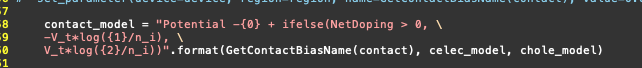# Simulation of the example diode

Hi, I am now studing DEVSIM and have read the documents and examples therein. It looks pretty well and the modelling way really impresses me. However, I have the following problems with respect to the script: examples/diode/diode_2d.py.

1. What‘s the result obtained by this script. My meaning is, the potential, carrier distribution and current vary with time, but the returned result is only at one time instant (The default tdelta in solve command is 0 by default). Thus I wonder the returned result is at which time instant? Steady state? Or at t = 0?

2. If I apply a voltage of 2V on the contact, and want to know the potential、carrier distribution、current at an arbitrary time t, then how should I use the codes? By using command like this?

tNow = 0
while (v<2.0){
set_parameter(device=device, name=GetContactBiasName(“top”), value=v)
solve(type=“dc”, absolute_error=absTol, relative_error=reltol, tdelta = DeltaT)
v += 0.1
tNow += DeltaT
}

set_parameter(device=device, name=GetContactBiasName(“top”), value=2)
while (tNow<t){
solve(type=“dc”, absolute_error=absTol, relative_error=reltol, tdelta = DeltaT)
tNow += DeltaT
}

1. If I want to solve another 2D Diode problem, how should I modify the script? From my understanding, all I need is to modify “diode_common.Create2DMesh”, “diode_common.SetParameters”, “diode_common.SetNetDoping” and “simple_physics”.

Thanks a lot!

@cstg02, thank you for your interest in the software. Are you interested in a series of steady state dc solutions, or are you interested in the transient response?

1. The diode_2d.py script is meant to do basic tests of the simulator. The first loop:
####
#### Ramp the bias to 0.5 Volts
####
v = 0.0
while v < 0.51:
set_parameter(device=device, name=GetContactBiasName("top"), value=v)
solve(type="dc", absolute_error=1e10, relative_error=1e-10, maximum_iterations=30)
PrintCurrents(device, "top")
PrintCurrents(device, "bot")
v += 0.1


is meant to ramp a series of steady state biases from 0.0 to 0.5 volts. The remainder of the script is testing error handling, if the bias step is to large, and the loop is used to reduce the bias step in the simulation.

1. Please modify the loop I have pasted above, and change the loop from testing 0.51 volts, to testing 2.01 volts. 2 volts is an extremely high bias for a silicon diode, so I do not think a result would be physical.

2. You can create your own diode using the internal mesher, as is done in diode_common.Create2DMesh, or you can create a mesh externally using Gmsh. If you need assistance with another meshing program format, please let me know. The other places you mention would be good to modify the simulation script.

Currently, I am mainly interested in the steady state simulation. The reason for my asking transient response is for the following reasons:

1. It would be potentially great if I could use DEVSIM to simulate transient states.
2. Since the manual for this example refers to the paper [SG69], while there is time advanced step in that paper, thus I tend to believe there might be something done with the ‘‘time’’.(Of course, steady state can be obtained by setting d/dt J = 0.) Furthermore, tdelta in the parameters of the solve function, and time_node_model in the equation establishment of diode example further strength this thought. So to fully understand the usage and principle of this example, I ask this question.
3. To test DEVSIM, I previously compare with Crosslight, using a different diode example, with different shape, doping, mobility, carrier lifetime(tau_n,tau_p). But the results differ. I believe the ability of DEVSIM to simulate this problem, and thus believe something went wrong for my usage.

It seems that my setting is correct. Thus it is strange why the results differ(In fact, the built-in voltage has already differed). Is it that my test example is non-physics? And Crosslight and DEVSIM solves different mathematic equations? The followings are the settings for the simulated test example:

Domain: [0,5e-6] x [0,5.5e-6] for Si
Doping: [0,2.5e-6] x [4e-6,5.5e-6] as acceptor(2e20), other parts doping as donor(2e20).
contacts: [0,1.5e-6] x [5.5e-6,5.5e-6] (0 V) and [0,5e-6] x [0,0] (-2 V).
Relative permittivity: 11.9.
Mobility: 0.13864.
tau_n=tau_p = 1e-8.
ni = 1.08e10.

The final purpose of my using DEVSIM will be simulating a more compliciated device, e.g., LDMOS, HEMT.

It seems that the main effort in using DEVSIM is to find the suitable mathematical equations for regions, interfaces and contacts, which I am now searching for. This seems to be a difficult work. But once they have been searched, DEVSIM is flexible enough to solve these problems, which is the main reason I am so interested in DEVSIM.

It might be good for me to provide some details between the difference between DEVSIM and Crosslight. The results of DEVSIM and Crosslight have the similar shapes, but the values differ. In fact, the built-in voltage has already differred greatly, which makes the final potential range(i.e., max potential minus min potential) be limited to about 0.7v in DEVSIM, while it is about 1.5v in Crosslight.

Hi @cstg02 , thank you for your interest in the software. For the basic device physics, the potential is tied to the middle of the bandgap E_i. Please see python_packages/simple_physics.py in the routine CreateSiliconPotentialOnlyContact.Or

\psi - V_{top} - V_T \log \left( n_c / n_i \right)

for an electron-doped Ohmic contact with:

n_c = (10^{-10} + 0.5*\mathrm{abs}(N_D+ \left(N_D^2 + 4 * n_i^2\right)^{0.5}))

and similar relations for a accepter-doped contact.

Please note that the length unit in these scripts are cm, including in the coordinates positions for the mesh. What unit are you using for a mobility of 0.13864?